Carbonate Species Distribution; Alkalinity

Let is suppose that we have put CT millimoles of carbo into 1 liter of water. This could be CT millimoles of carbonic acid, CT millimoles of bicarbonate ion or CT millimoles of carbonate ion or  CT/3 millimoles of each of the three or any combination such that there are CT millimoles of carbon atoms (each of the 3 species contains 1 carbon atom per molecule or ion) in the solution in any or all of these species. The species will distribute themselves such that there are f1CT millimoles of carbonic acid, f2Cof bicarbonate ion and f3Cof carbonate ions. f+ f2  + f3 = 1.  The f's depend on the pH of the solution and on the pK's of carbonic acid which are, respectively, pK1 = 6.38 and pK2 = 10.38 both at 20 °C.  These two numbers are, respectively, the negative logarithms of the equilibrium contsants associated with, respectively, the first and second dissociations of carbonic acid which are described in the article on chalk.   The f's are simply calculated as follows: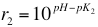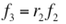A graph showing the values of the f's as a function of pH can be seen in the article on measuring alkalinity'.

Henderson - Hasselbalch Equation

The relationships just given are derived from the Henderson-Hasselbalch equation which is a form of the law of mass action. The dissociation of carbonic acid which yields a hydrogen ion (proton) and a bicarbonate ion is written:The Law of Mass Action, as applied to this equation,gives the ratios of the concentrations at equilibrium by the bracketed items (which are really really activites which, for dilute solutions, are very close to concentrations), and K1 is the equilbrium constant for this reaction. The p operator is defined byIn words: to apply the p operator to a quantity one takes the logarithm to the base 10 and multiplies the result by -1. Applying the p operator to all elements of the mass action equation we get: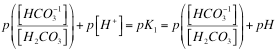Note that the p operator acting on the hydrogen ion activity returns the pH. The inverse of the p operator,p-1, is, in words: multiply the operand by -1 and take the antilogarithm. The antilogarithm is found by raising 10 to the power of the argument. p-1(p(X)) = X so that applying p-1 to both sides and rearranging we getCharge

If we have f1CT millimoles of carbonic acid, f2Cof bicarbonate ion and f3Cof carbonate ions then the total charge on the ions must be: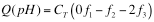because the charge on each carbonic acid molecule is 0, on each bicarbonate ion is -1 and on each carbonate ion, -2. Note that Q is a function of pH because each f is a fucntion of pH. If Cis the number of millimoles per liter then Q will be the total charge on all carbo species per liter in mEq/L.

Alkalinity

The alkalinity of water is defined as the number of mEq of protons (acid) which must be added to 1 liter of a sample to cause its pH to come to some target pH. When measuring alkalinity in the lab the target pH is usually the methyl-orange end point pHIf a water sample has sample pHwith total carbo per liter is equal to CT and we add acid to a 1 liter sample of it until  pHM is reached then we will have changed the charge on the liter's carbo species from CTQ(pHS) to CTQ(pHM). The amount of acid required to do that isThis is not the total alkalinity, however as it is not only the carbo that needs to be shifted but the pH of the water itself. At pHS the concentrations of hydrogen ions and hydroxyl ions areAt pHM the concentrations of hydrogen ions and hydroxyl ions are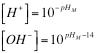Increasing the hydrogen ion concentration requires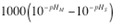mmol/L extra protons because the proton concentration in the solution is higher at pHM with the factor of 1000 being necessary becauseare both in moles per liter and alkalinity is specified in millimoles. Similarly decreasing the hydroxyl ion content at pHto the lower level at  pHrequires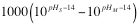mEq/L more  because each hydroxyl ion anihilated takes 1 proton: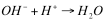We could call this second demand for protons the alkalinity of the water itself: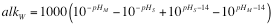For the M alkalinity end point and typical sample pH the alkalinity of the water alone is around 2.5 ppm as CaCO3.

The whole alkalinity is the sum of the carbo alkalinity and the water alkalinity:This, of course, assumes that the only proton absorbing substances in the water under consideration are carbonates and the water itself. If, for example, the water contains phosphate a set of similar reactions with the various protonation states of phosphate ions takes place and the alkalinity would have to include an alkCP term to account for that. When we are given a water report we are given the alkalinity and pHS. We also need to know the end point , pHM, used when the alkalinity was measured. Assuming that we have that we can then solve the eqation above for CT.The individual concentrations of carbonic, bicarbonate and carbonate can, given CT, be easily calculated from their fractions.